# Precalculus : Find the Zeros of a Function Using the Rational Zeros Theorem

## Example Questions

### Example Question #1 : Integral And Rational Zeros Of Polynomial Functions

Use the Rational Zero Theorem to find all potential rational zeros of the polynomial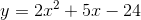. Which of these is NOT a potential zero?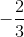Explanation:

To find the potential rational zeros by using the Rational Zero Theorem, first list the factors of the leading coefficient and the constant term:

Constant 24: 1, 2, 3, 4, 6, 8, 12, 24

Now we have to divide every factor from the first list by every factor of the second: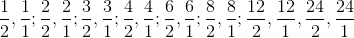Removing duplicates [for example,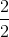and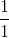are both equivalent to 1] gives us the following list: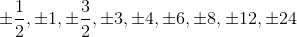The only choice not on this list is.

### Example Question #512 : Pre Calculus

Consider the polynomial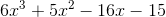. Of the potential rational zeros provided by the Rational Zero Theorem, which can we determine to NOT be a solution?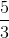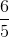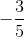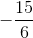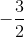Explanation:

The potential zeros must have a factor of -15 as their numerator and a factor of 6 as their denominator. This eliminatesas a possibility since 6 is not a factor of -15.

Now we need to test which of these values actually give zero when plugged into the polynomial.

First,: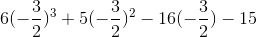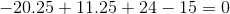Now: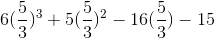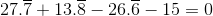Finally: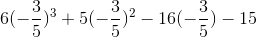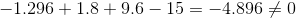Since this one doesn't give us zero, it is not a solution of the polynomial.

### All Precalculus Resources